# Parabola facts for kids

Kids Encyclopedia Facts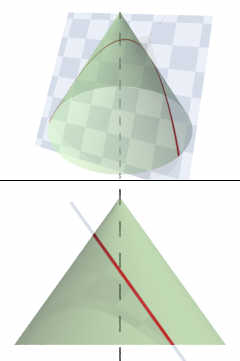A parabola obtained as the intersection of a cone with a plane parallel to a straight line on its surface

The parabola (from the Greek παραβολή) is a type of curve. Menaechmus (380–320 BC) discovered the parabola, and Apollonius of Perga (262 BC–c190 BC) first named it.

A parabola is a conic section. If a cone is dissected by a plane which is parallel to one of the surfaces of the cone, the result is a parabola. The point where the parabola reaches its maximum or minimum is called the "vertex." At this point the curvature of the parabola is greatest.

Each parabola has a focal point. Any ray that enters the parabola and is parallel to the axis of symmetry will pass through this point after being reflected by the curve. Because of this fact, parabolas are important in devices such as satellite dishes, or magnifying mirrors. Parabolas are often used to approximate curves that are more difficult to model by themselves.

Every parabola uses the equation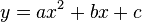$y = ax^2 + bx + c$, where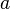$a$,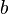$b$ and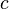$c$ are constants, and$a$ is not equal to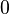$0$.

## Images for kidsParabola Facts for Kids. Kiddle Encyclopedia.